Home > A2C > Chapter 7 > Lesson 7.2.1 > Problem7-97

7-97.
1. Simplify each expression below. If you are stuck, the ideas in problem 7-74 should be helpful. Homework Help ✎

1.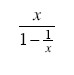2.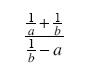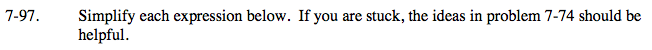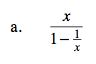Use a Giant One to eliminate the denominator of the fraction in the denominator.

Distribute:

$\frac{x}{x} \cdot \frac{x}{1-\frac{1}{x}}$

$\frac{1}{x} \cdot x = 1$

$\frac{x^2}{x-1}$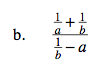Eliminate all the denominators of the smaller fractions. What will the Giant One look like?

Distribute:

$\frac{ab}{ab} \cdot \frac{ \frac{1}{a} - \frac{1}{b} }{ \frac{1}{b} -a}$